# Measurement Notes

B-dot Probes:  These solenoid coils and loop probes are known as "B-dot" sensors or probes since the voltage output is the time-derivative of the magnetic field.  This is based on Faraday's Law which says the electromotive force around a closed loop is equal to the negative of the time rate of change of the magnetic flux enclosed by the loop:  e = -dF/dt.  The following oscilloscope screenshots show some examples.

The violet traces (top curve) in all the following screenshots is proportional to the magnetic field, since it plots the current through the Helmholtz coil which produces the field. The blue traces (bottom curve) in the figures is the output voltage from the B-dot sensor placed in the magnetic field. The horizontal axis is time.

Figure 1. Top curve (violet) shows a Sine wave (CW) magnetic field.  Bottom curve (blue) shows resulting Cosine output voltage at the same frequency from B-dot sensor. The two horizontal red dotted lines shows the zeros and peaks of the Cosine, the two vertical red dotted lines shows zeros of the Cosine or Sine. Frequency is 150 Hz, using resonant sensor MC95Rw. All sensor models can be used to measure sinusoidal fields.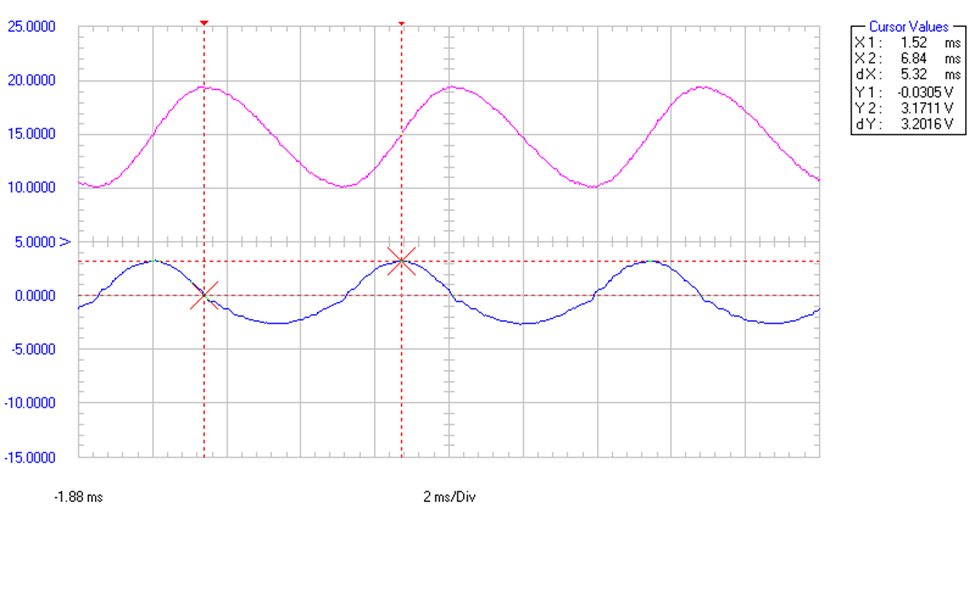To calculate the magnetic field strength in Gauss of the sine wave field shown above in Figure 1: the B-dot sensor output (blue curve) has amplitude of 3 V, so consulting the graph at top of magneticsciences.com/magnetic-field-sensors for sensor model MC95Rw at frequency 150 Hz shows 5.4 Volts per Gauss, so 3V divided by 5.4 Volts per Gauss shows the magnetic field amplitude of the sine wave field was 0.55 Gauss.

A 180 degree phase reversal for the output voltage may be due to the negative sign in Faraday's law. Also additional phase reversals may result from flipping the sensor around so its oriented the opposite direction in the field, or the polarity of the internal coil wire connection in the sensor, or pushing the 'invert’ button for the scope channel, or it can result from the trigger setting for the scope trace.

Figure 2. Top curve (violet) shows a Square wave magnetic field. The bottom curve (blue) is the resulting output voltage from B-dot probe model MC95A. The large fast rise and fall times of the square wave field (violet) causes a bump in the first derivative, as seen in the B-dot sensor voltage output (blue). Fundamental frequency is 150 Hz, using non-resonant sensor MC95A.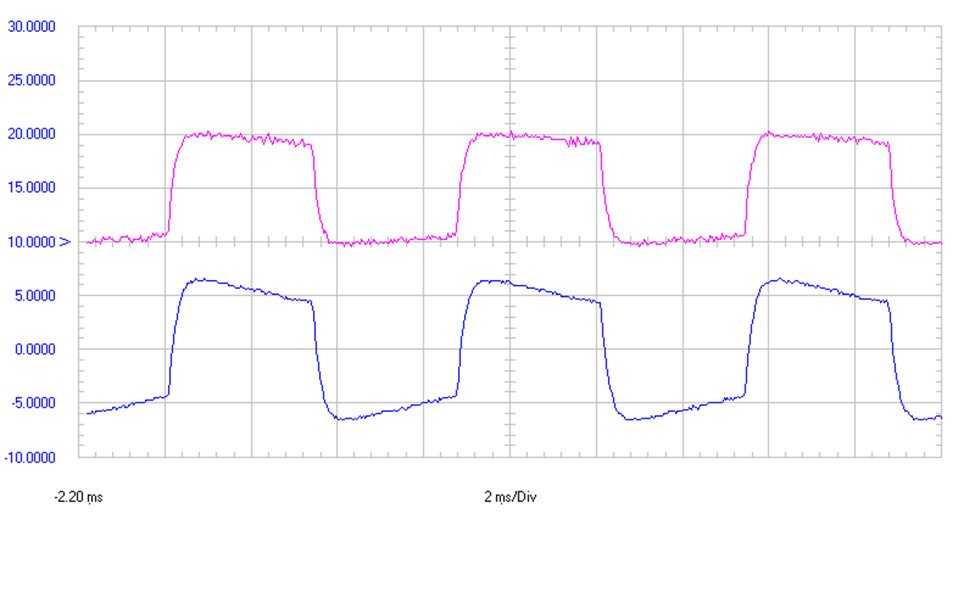Calculating the field strength of non-sinusoidal fields using the MC series sensors would be approximate, and best to use RMS values of the voltage to compute the RMS value of the field, to minimize the effect of voltage peaks or noise.

Figure 3. Top curve (violet) is a magnetic field with very rapid large changes. Lower curve (blue) is the B-dot sensor output, it shows peaks whenever the magnetic field shows rapid large changes, due to large first derivative of the field.  Fundamental frequency is 15 Hz, using non-resonant sensor MC95A.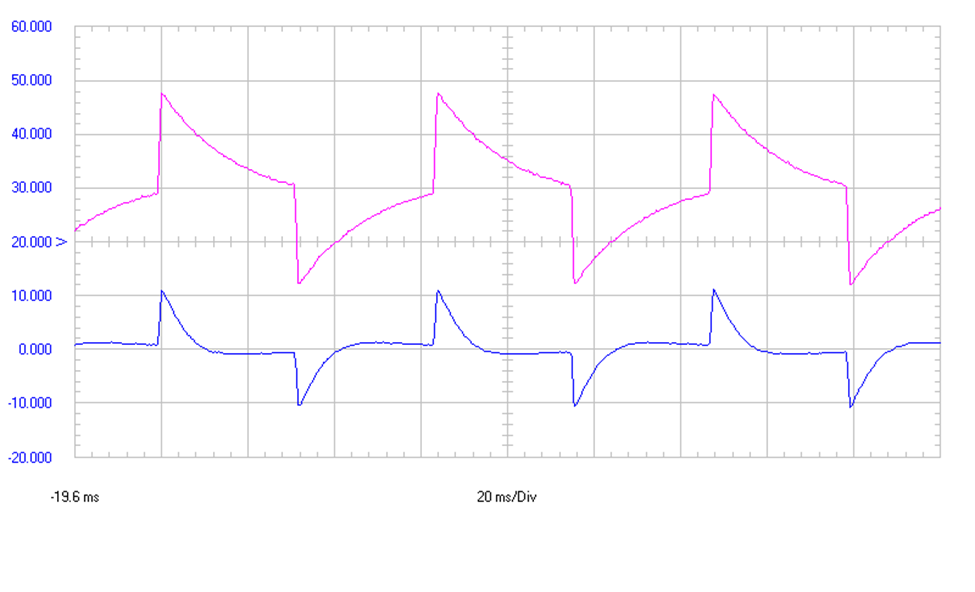The derivative of a square wave field is alternating negative and positive peaks. The derivative of a triangular field waveform is constant low-value of alternating polarity as the field ramps up and down. The derivative of a sawtooth field is constant low value as the field ramps slowly up, then a peak of the other polarity as the sawtooth field rapidly drops.

On a spectrum analyzer you would see the Fourier frequency components of the output voltage from the sensor. If your instrument can display frequency, you can read the predominant frequency. You can also use the sensor with a data logger that accepts volts at your frequency.

Figure 4 top curve (violet) shows a square wave field. The bottom curve (blue) shows resulting voltage output from a resonant sensor.  It is seen that the resonant sensor output starts ringing each time the square wave field changes abruptly. The ringing is due to exposure to frequency components at the resonant frequency contained within the fast rise and fall of the square wave field. Fundamental frequency is 150 Hz, using resonant sensor MC95Rw.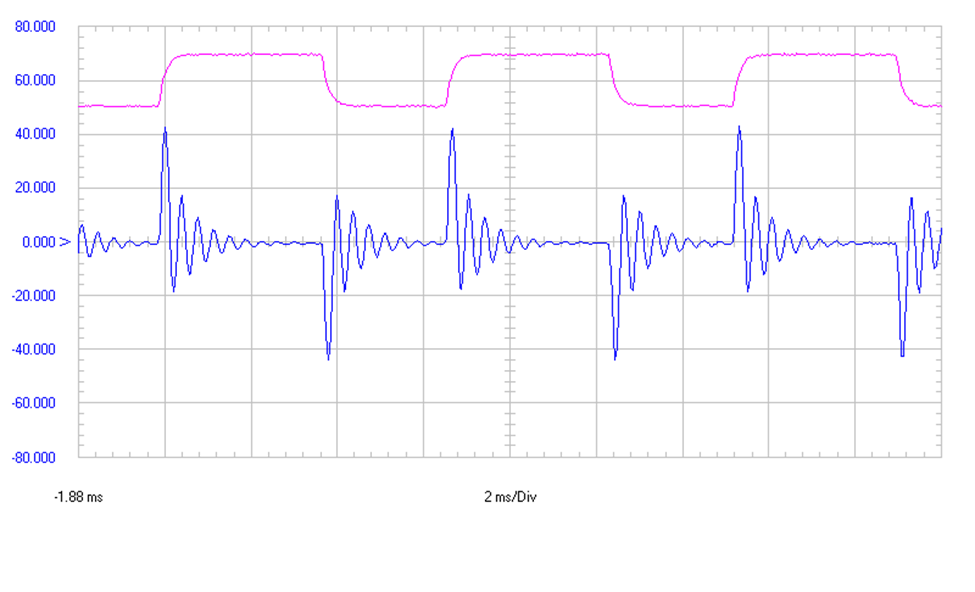General Measurement Notes:

B-dot sensors can measure magnetic fields over a range of frequencies, but they do not measure static (DC) magnetic fields.

A single-axis sensor measures magnetic fields parallel to the sensor axis. Therefore, rotate the sensor to all pointing directions (while keeping the sensor at the location you want to measure). When you see the maximum output, then the sensor is parallel to the maximum magnetic field polarization. Then use the curves or calibration data to determine the maximum field at that location.

The entire length of the MC sensor is used to make the measurement. Therefore, if the field is not uniform (not the same) over the length of the MC sensor, then the output will show the approximate average over the length of the sensor. So it is suggested that you center the MC sensor at the location you want to measure. The closer you measure to the source, the more non-uniform the field will generally be. The farther away, the more uniform the field will be.

The voltage output from MC sensor can be measured as RMS voltage or as Peak voltage. RMS voltage measurements can reduce the effect of noise or other peaks on the measurement. If your display instrument is measuring RMS voltage then you are measuring RMS magnetic field strength, or if measuring Peak voltage then you are measuring Peak magnetic field strength (of a sinusoidal CW field).

A possible cause of measurement error is if your connecting cables or other test equipment is radiating or picking up electromagnetic fields. This is not desired, since ideally the test equipment and cables should not be radiating or picking up any fields, only your selected source of the fields should be radiating, and only the sensor receiving. This problem may be noticed if moving the cables or equipment causes changes in the output voltage (while keeping the source and the sensor unmoved).

Reflections from the room and equipment, and metal table, shelves, chairs, etc near the test area may also affect the measured field.  Especially, using an MC series sensor in close proximity to steel or other ferromagnetic material can perturb the magnetic field which affects the accuracy of the calibration data and can worsen the cross-polarization response. For the same reason, if using more than one MC series sensor in very close proximity to each other, it is recommended to mount the sensors at least 1 sensor length away from each other to reduce perturbations due to nearby ferrites in the other sensors.

Sharp bending or yanking of your coaxial cable might break the wires inside the coax, which is usually seen as erratic readings. If a sensor seems broken it may be due to an intermittent coaxial connector, or broken coax center conductor due to sharp bending. In other cases the coil inside the sensor may have been damaged due to a strong magnetic field which changed rapidly, producing a large voltage spike at the sensor output.

If using a series resistor to measure current into your source, note that some resistor types are only accurate at lower frequencies.  The resistor should be a type that does not have a large error in resistance at the frequencies being tested. The voltages measured (across said resistor, and the voltage output from MC sensor) should be both be measuring RMS voltage, or both measuring Peak voltage, (not one RMS and the other Peak), to use for input to measurement calculations.

Your source of magnetic field (for example Helmholtz coil or a loop) may need input from a generator or oscillator, via coaxial cable or often via 'balanced' input from two-wire lines or from a balun. If using two-wires then a twisted pair can help reduce radiation from the wires.

In elliptically polarized fields the maximum reading of the sensor will be in the polarization direction of the major axis of the polarization ellipse. Therefore these single-axis sensors avoid a large error seen with most triple-axis meters when measuring elliptically or circularly polarized fields (or near 3-phase power lines), due to the sequential (not simultaneous) sampling of X,Y,Z field polarizations done by most triple-axis meters.

1/r2  Field Roll-off is often seen

In many situations the field is inversely proportional to the range squared from the emitter:   1/r2 .

For example: at 27 kHz the voltage output Vo was measured from an MC161 sensor placed along the Z axis of a small loop to measure Br the radially polarized field, at two distances:   ra=80 cm and rb=100cm.  The ratio of these two ranges  r/ rb   inverted and squared = 1002/802 = 1.56

The measured sensor output voltage at ra was Vo = 5.9V,  and at rb it was Vo = 3.8V.   So the ratio of the voltages at  r/ rb  = 5.9/3.8 = 1.55   which is almost exactly equal to the inverted range squared proportionality of 1.56

Magnetic field flux density B emitted by a small loop:

Below is shown the equations for the magnetic field flux density B radiated by a small loop or multiturn loop in spherical coordinate system. This field is also similar to the field radiated by many types of small magnetic field sources which resemble loop currents. In the diagram the loop lies in the XY plane with axis along the Z axis. The range to observation point is

Br is the radially polarized magnetic field flux density, with maximum on axis of loop (at q= 0°), minimum in plane of loop (at q = 90°)

Bq is theta q polarized magnetic flux density (along theta vector), maximum is in plane of loop (at q = 90°), and minimum on axis of loop (q = 0°).

(the third polarization component Bf is not emitted nor received by a small loop).

Beta  b = 2p/l  is the "phase constant".

m = INA  is “Magnetic moment” where A is the area of the loop, N is number of turns of the loop, and I is the current in loop wire.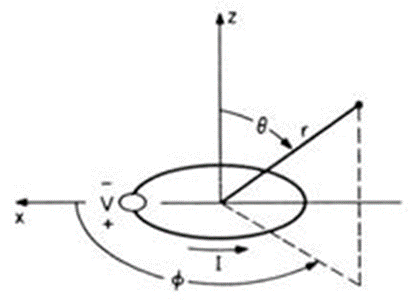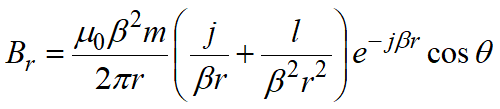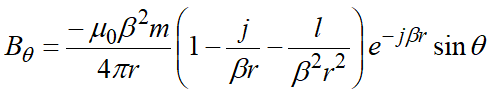For Br equation: bringing the 1/r inside parenthesis: if r is very small 1/b2r 3 is the largest term, so fields diminish as 1/r3  very close to the loop.

As r is increased, the largest term is 1/br2 so there the field is inversely proportional to r2 ,  it is usually the largest term in Br

Bq has a different equation as is shown above, so it may decline more gradually vs distance due to a 1/r term